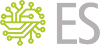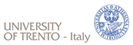# MathSAT 5An SMT Solver for Formal Verification & More

## MathSAT Low-level API Reference

Low-level API for the MathSAT SMT solver. More...

## Data Structures

struct  msat_ll_solver
Low-level inteface to the MathSAT DPLL(T) solver.

struct  msat_satproof
Low-level interface to SAT resultion proofs.

## Macros

#define msat_satvar_undef   ((msat_satvar)0)
special undefined SAT var More...

#define msat_satlit_undef   ((msat_satlit)0)
special undefined SAT lit More...

#define msat_make_satlit(var, neg)   ((neg) ? -(var) : (var))
literal creation from a variable and a sign More...

#define msat_satlit_var(lit)   ((lit) < 0 ? -(lit) : (lit))
get the variable corresponding to lit More...

#define msat_satlit_negated(lit)   ((lit) < 0)
check whether the literal is negated More...

#define msat_satlit_negate(lit)   (-(lit))
negate a literal More...

#define MSAT_ERROR_LL_SOLVER(s)   ((s).repr == NULL)
Error checking for msat_ll_solver. More...

#define MSAT_ERROR_SATPROOF(s)   ((s).repr == NULL)
Error checking for msat_satproof. More...

## Typedefs

typedef int32_t msat_satvar
SAT solver variables. More...

typedef int32_t msat_satlit
SAT solver literals. More...

## Functions

msat_ll_solver msat_create_ll_solver (msat_env env, int tsolver)
Create a low-level DPLL(T) solver. More...

int msat_destroy_ll_solver (msat_ll_solver s)
Destroys a low-level DPLL(T) solver. More...

msat_satvar msat_ll_make_var (msat_ll_solver s)
Create a new variable in the SAT solver. More...

msat_satvar msat_ll_make_proxy_var (msat_ll_solver s, msat_term atom)
Create a proxy variable for the given theory atom. More...

int msat_ll_set_var_polarity (msat_ll_solver s, msat_satvar v, msat_truth_value pol)
sets the polarity used when branching on the variable v More...

int msat_ll_set_var_decision (msat_ll_solver s, msat_satvar v, int yes)
Enable/disable branching on v. More...

int msat_ll_add_preferred_var (msat_ll_solver s, msat_satvar v)
Adds a variable at the end of the list of preferred variables for branching when solving the problem. More...

int msat_ll_clear_preferred_vars (msat_ll_solver s)
Clears the list of preferred variables for branching. More...

msat_term * msat_ll_encode_constraints (msat_ll_solver s, msat_term term, size_t *out_n)
Generate a list of additional axioms encoding unsupported functions/predicates in term. More...

int msat_ll_begin_clause (msat_ll_solver s)
starts a new clause in the solver More...

int msat_ll_addlit (msat_ll_solver s, msat_satlit l)
adds a literal to the current clause in the solver More...

int msat_ll_end_clause (msat_ll_solver s)
Ends the current clause in the solver. More...

int msat_ll_push_backtrack_point (msat_ll_solver s)
Pushes a checkpoint for backtracking in the solver. More...

int msat_ll_pop_backtrack_point (msat_ll_solver s)
Backtracks to the last checkpoint set in the solver. More...

msat_result msat_ll_solve (msat_ll_solver s, msat_satlit *assumptions, size_t num_assumptions)
Checks satisfiability under the given assumptions. More...

char * msat_ll_get_search_stats (msat_ll_solver s)
Returns a string with search statistics for the given solver. More...

int msat_ll_is_unsat_assumption (msat_ll_solver s, msat_satlit l)
Tests whether the given assumption is part of the unsatisfiable core. More...

msat_truth_value msat_ll_get_model_value (msat_ll_solver s, msat_satvar v)
Retrieves the truth assignment for the given variable in the solver. More...

int msat_ll_build_theory_model (msat_ll_solver s)
Asks the solver to construct a theory model. More...

msat_term msat_ll_get_theory_model_value (msat_ll_solver s, msat_term v)
Retrieves the model value for the given term. More...

msat_satproof msat_ll_get_unsat_proof (msat_ll_solver s)
Retrieves the resolution proof of unsatisfiability computed by the solver. More...

int msat_satproof_is_res (msat_satproof p)
Checks whether the given proof is a resolution chain. More...

int msat_satproof_res_arity (msat_satproof p)
Returns the arity of the given resolution chain. More...

int msat_satproof_res_step (msat_satproof p, int idx, msat_satlit *pivot, msat_satproof *c)
Retrieves the step at index idx of the given resolution chain. More...

int msat_satproof_clause_arity (msat_satproof p)
Retrieves the arity of the clause attached to the given leaf proof. More...

msat_satlit msat_satproof_clause_lit (msat_satproof p, int idx)
Retrieves the literal at the given index in the clause attached to the given leaf proof. More...

## Detailed Description

Low-level API for the MathSAT SMT solver.

## Macro Definition Documentation

 #define MSAT_ERROR_LL_SOLVER ( s ) ((s).repr == NULL)

Error checking for msat_ll_solver.

 #define MSAT_ERROR_SATPROOF ( s ) ((s).repr == NULL)

Error checking for msat_satproof.

 #define msat_make_satlit ( var, neg ) ((neg) ? -(var) : (var))

literal creation from a variable and a sign

 #define msat_satlit_negate ( lit ) (-(lit))

negate a literal

 #define msat_satlit_negated ( lit ) ((lit) < 0)

check whether the literal is negated

 #define msat_satlit_undef   ((msat_satlit)0)

special undefined SAT lit

 #define msat_satlit_var ( lit ) ((lit) < 0 ? -(lit) : (lit))

get the variable corresponding to lit

 #define msat_satvar_undef   ((msat_satvar)0)

special undefined SAT var

## Typedef Documentation

 typedef int32_t msat_satlit

SAT solver literals.

 typedef int32_t msat_satvar

SAT solver variables.

## Function Documentation

 msat_ll_solver msat_create_ll_solver ( msat_env env, int tsolver )

Create a low-level DPLL(T) solver.

Configuration parameters are read from the parent environment

Parameters
 env The parent environment tsolver If nonzero, enable support for theories, otherwise a pure SAT solver is created
Returns
the created SAT solver. In case of errors, a solver s.t. MSAT_ERROR_SATSOLVER(solver) is true is returned
 int msat_destroy_ll_solver ( msat_ll_solver s )

Destroys a low-level DPLL(T) solver.

Parameters
 s The solver to destroy
Returns
zero on success, nonzero on error
 int msat_ll_add_preferred_var ( msat_ll_solver s, msat_satvar v )

Adds a variable at the end of the list of preferred variables for branching when solving the problem.

The polarity can be controlled with msat_ll_set_var_polarity()

Parameters
 s The solver in which to operate v The variable to add to the preferred list
Returns
zero on success, nonzero on error
 int msat_ll_addlit ( msat_ll_solver s, msat_satlit l )

adds a literal to the current clause in the solver

Parameters
 s The solver in which to operate l The literal to add
Returns
zero on success, nonzero on error
 int msat_ll_begin_clause ( msat_ll_solver s )

starts a new clause in the solver

Parameters
 s The solver in which to operate
Returns
zero on success, nonzero on error
 int msat_ll_build_theory_model ( msat_ll_solver s )

Asks the solver to construct a theory model.

Parameters
 s The solver in which to operate
Returns
zero on success, nonzero on error
 int msat_ll_clear_preferred_vars ( msat_ll_solver s )

Clears the list of preferred variables for branching.

Parameters
 s The solver in which to operate
Returns
zero on success, nonzero on error
 msat_term* msat_ll_encode_constraints ( msat_ll_solver s, msat_term term, size_t * out_n )

Generate a list of additional axioms encoding unsupported functions/predicates in term.

Each term in the returned array is an additional axiom that should be added to s. Note that axioms can be arbitrary formulas, and so must be converted to CNF to be added to the solver.

Calling this function is necessary for correctness whenever term contains subterms with the following tags:

• MSAT_TAG_TERM_ITE
• MSAT_TAG_INT_MOD_CONGR
• MSAT_TAG_FLOOR
• MSAT_TAG_INT_TO_BV
• MSAT_TAG_INT_FROM_UBV
• MSAT_TAG_INT_FROM_SBV
Parameters
 s The solver in which to operate term The term containing the constraints to encode out_n Output parameter for storing the size of the returned array. Must not be NULL
Returns
an array of additional axioms to add to the solver, or NULL in case of error. The array must be deallocated by the user with msat_free()
 int msat_ll_end_clause ( msat_ll_solver s )

Ends the current clause in the solver.

Parameters
 s The solver in which to operate
Returns
zero on success, nonzero on error
 msat_truth_value msat_ll_get_model_value ( msat_ll_solver s, msat_satvar v )

Retrieves the truth assignment for the given variable in the solver.

Parameters
 s The solver in which to operate v A variable
Returns
The truth assignment for v in s, or MSAT_UNDEF in case of errors (or if the value is actually unknown)
 char* msat_ll_get_search_stats ( msat_ll_solver s )

Returns a string with search statistics for the given solver.

The returned string will contain newline-separated statistics for the DPLL engine and the active theory solvers (if any).

Parameters
 s The solver in which to operate.
Returns
A string with search statistics, or NULL in case of errors. The string must be deallocated by the oser with msat_free().
 msat_term msat_ll_get_theory_model_value ( msat_ll_solver s, msat_term v )

Retrieves the model value for the given term.

Model generation must be enabled in the environment passed to msat_create_ll_solver(). Before invoking this function, you must call msat_ll_build_theory_model()

Parameters
 s The solver in which to operate v A term
Returns
The model value for v in s, or a t s.t. MSAT_ERROR_TERM(t) is true in case of errors
 msat_satproof msat_ll_get_unsat_proof ( msat_ll_solver s )

Retrieves the resolution proof of unsatisfiability computed by the solver.

Proof generation must be enabled in the environment passed to msat_create_ll_solver()

Parameters
 s The solver in which to operate
Returns
the resolution refutation, or a proof p s.t. MSAT_ERROR_SATPROOF(p) is true in case of errors
 int msat_ll_is_unsat_assumption ( msat_ll_solver s, msat_satlit l )

Tests whether the given assumption is part of the unsatisfiable core.

Parameters
 s The solver in which to operate l The assumption to test
Returns
1 if l is in the unsat core, 0 if not, -1 on error
 msat_satvar msat_ll_make_proxy_var ( msat_ll_solver s, msat_term atom )

Create a proxy variable for the given theory atom.

Note that this does not add constraints for encoding unsupported functions/predicates automatically. In order to ensure correctness, you must manually call msat_ll_encode_constraints()

Parameters
 s The solver in which to operate atom The atom to proxy
Returns
A proxy variable for atom, or msat_satvar_undef on error
 msat_satvar msat_ll_make_var ( msat_ll_solver s )

Create a new variable in the SAT solver.

Parameters
 s The solver in which to operate
Returns
The new variable, or msat_satvar_undef on error
 int msat_ll_pop_backtrack_point ( msat_ll_solver s )

Backtracks to the last checkpoint set in the solver.

Parameters
 s The solver in which to operate
Returns
zero on success, nonzero on error
 int msat_ll_push_backtrack_point ( msat_ll_solver s )

Pushes a checkpoint for backtracking in the solver.

Parameters
 s The solver in which to operate
Returns
zero on success, nonzero on error
 int msat_ll_set_var_decision ( msat_ll_solver s, msat_satvar v, int yes )

Enable/disable branching on v.

Parameters
 s The solver in which to operate v The variable to set the branching yes If nonzero, enable branching on this variable
Returns
zero on success, nonzero on error
 int msat_ll_set_var_polarity ( msat_ll_solver s, msat_satvar v, msat_truth_value pol )

sets the polarity used when branching on the variable v

If pol is MSAT_UNDEF, the default polarity will be used

Parameters
 s The solver in which to operate v The variable to set the polarity pol The polarity value
Returns
zero on success, nonzero on error
 msat_result msat_ll_solve ( msat_ll_solver s, msat_satlit * assumptions, size_t num_assumptions )

Checks satisfiability under the given assumptions.

Parameters
 s The solver in which to operate assumptions An optional list of assumption literals (can be NULL) num_assumptions The size of the assumption array
Returns
MSAT_SAT if the problem is satisfiable, MSAT_UNSAT if it is unsatisfiable, and MSAT_UNKNOWN if there was some error or if the satisfiability can't be determined.
 int msat_satproof_clause_arity ( msat_satproof p )

Retrieves the arity of the clause attached to the given leaf proof.

Parameters
 p A leaf proof
Returns
the clause arity, or -1 on error
 msat_satlit msat_satproof_clause_lit ( msat_satproof p, int idx )

Retrieves the literal at the given index in the clause attached to the given leaf proof.

Parameters
 p A leaf proof idx The index of the literal to retrieve
Returns
the literal at the given index, or msat_satlit_undef on error
 int msat_satproof_is_res ( msat_satproof p )

Checks whether the given proof is a resolution chain.

Parameters
 p A resolution proof
Returns
1 of the proof is a resolution chain, 0 if not, -1 on error
 int msat_satproof_res_arity ( msat_satproof p )

Returns the arity of the given resolution chain.

Parameters
 p A resolution chain
Returns
the arity of p, or -1 on error
 int msat_satproof_res_step ( msat_satproof p, int idx, msat_satlit * pivot, msat_satproof * c )

Retrieves the step at index idx of the given resolution chain.

A step consists of a pair (pivot_literal, right_branch), where the left branch is the current proof p.

Parameters
 p A resolution chain idx The index of the step to retrieve pivot Output parameter for the pivot. If idx is zero, the pivot is msat_satlit_undef c Output parameter for the right branch of the resolution
Returns
zero on success, nonzero on error
Contentsmathsat-announce mailing list